# Intermediate Geometry : How to find an angle in a pentagon

## Example Questions

### Example Question #1 : How To Find An Angle In A Pentagon

The angles at 3 verticies of a pentagon are 60, 80 and 100.  Which of the following could NOT be the measures of the other 2 angles?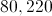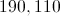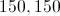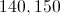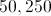Explanation:

The sum of the angles in a polygon is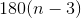For a pentagon, this equals 540. Since the first 3 angles add up to 240, the remaining 2 angles must add up to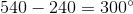### Example Question #2 : How To Find An Angle In A Pentagon

What is the sum of three angles in a hexagon if the perimeter of the hexagon is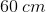?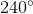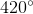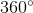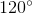Explanation:

The perimeter in this question is irrelevant. Use the interior angle formula to determine the total sum of the angles in a hexagon.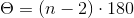There are six interior angles in a hexagon.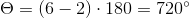Each angle will be a sixth of the total angle.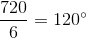Therefore, the sum of three angles in a hexagon is: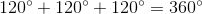### Example Question #3 : How To Find An Angle In A Pentagon

Add four interior angles in a regular pentagon. What is the result?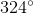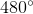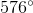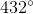Explanation:

Use the interior angle formula to find the total sum of angles in a pentagon.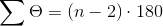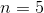for a pentagon, so substitute this value into the equation and solve: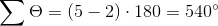Divide this number by 5, since there are five interior angles.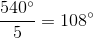The sum of four interior angles in a regular pentagon is: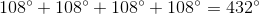### Example Question #4 : How To Find An Angle In A Pentagon

What is the sum of two interior angles of a regular pentagon if the perimeter is 6?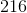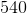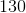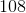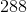Explanation:

The perimeter of a regular pentagon has no effect on the interior angles of the pentagon.

Use the following formula to solve for the sum of all interior angles in the pentagon.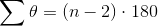Since there are 5 sides in a pentagon, substitute the side length.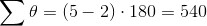Divide this by 5 to determine the value of each angle, and then multiply by 2 to determine the sum of 2 interior angles.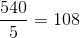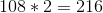The sum of 2 interior angles of a pentagon is.

### Example Question #5 : How To Find An Angle In A Pentagon

Suppose an interior angle of a regular pentagon is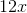.  What is?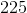Explanation:

The pentagon has 5 sides. To find the value of the interior angle of a pentagon, use the following formula to find the sum of all interior angles.Substitute.Divide this number by 5 to determine the value of each interior angle.Every interior angle is 108 degrees.  The problem states that an interior angle is.  Set these two values equal to each other and solve for.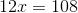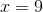### Example Question #6 : How To Find An Angle In A Pentagon

Let the area of a regular pentagon be.  What is the value of an interior angle?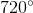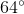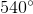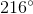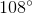Explanation:

Area has no effect on the value of the interior angles of a pentagon. To find the sum of all angles of a pentagon, use the following formula, whereis the number of sides:There are 5 sides in a pentagon.Divide this number by 5 to determine the value of each angle.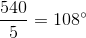### Example Question #7 : How To Find An Angle In A Pentagon

True or false: Each of the five angles of a regular pentagon measures.

True

False

False

Explanation:

A regular polygon withsides hascongruent angles, each of which measures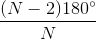Setting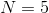, the common angle measure can be calculated to be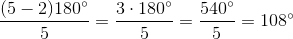The statement is therefore false.

### Example Question #8 : How To Find An Angle In A Pentagon

True or false: Each of the exterior angles of a regular pentagon measures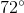.

True

False

True

Explanation:

If one exterior angle is taken at each vertex of any polygon, and their measures are added, the sum is. Each exterior angle of a regular pentagon has the same measure, so if we letbe that common measure, then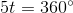Solve for: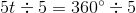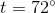The statement is true.

### Example Question #9 : How To Find An Angle In A Pentagon

Given: Pentagon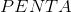.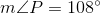True, false, or undetermined: Pentagonis regular.

True

Undetermined

False

Undetermined

Explanation:

Suppose Pentagonis regular. Each angle of a regular polygon ofsides has measureA pentagon has 5 sides, so set; each angle of the regular hexagon has measure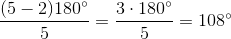Since one angle is given to be of measure, the pentagon might be regular - but without knowing more, it cannot be determined for certain. Therefore, the correct choice is "undetermined".